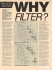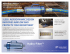# Matlab Based Design of Adaptive Filters Using Least Pth Norm: FIR

## Transcription

Matlab Based Design of Adaptive Filters Using Least Pth Norm: FIR
```International Journal of Signal Processing Systems Vol. 2, No. 1 June 2014
Matlab Based Design of Adaptive Filters Using
Least Pth Norm: FIR vs IIR
Srishtee Chaudhary
GPCG, ECE Dept, Patiala, Punjab, India
Email: [email protected]
Abstract—Adaptive filters are considered nonlinear systems;
therefore their behavior analysis is more complicated than
for fixed filters. As adaptive filters are self designing filters,
their design can be considered less involved than in the case
of digital filters with fixed coefficients. The paper discusses
optimization methods, algorithms for a filter, IIR and FIR
filter designs, in order to improve a prescribed performance
criterion. Further least Pth norm approach is compared for
both FIR and IIR filter. Since no specifications are available,
the adaptive algorithm that determines the updating of the
filter coefficients requires extra information that is usually
given in the form of a signal. This signal is in general called
a desired or reference signal, whose choice depends on the
application.
a data-driven approximation step. Adaptive filters self
learn. Adaptive filters require two inputs: the signal and a
noise or reference input. As the signal into the filter
themselves to achieve the desired result, such as
identifying an unknown filter or canceling noise in the
input signal. New coefficients are sent to the filter from
coefficient generator. The coefficient generator is an
adaptive algorithm that modifies the coefficients in
response to an incoming signal. In most applications the
goal of the coefficient generator is to match the filter
coefficient to the noise so the adaptive filter can subtract
the noise out from the signal. Since, the noise signal
changes the coefficients must vary to match it, hence the
name adaptive filters. Designing the filter does not
require any other frequency response information or
specification. To define the self-learning process, select
the adaptive algorithm used to reduce the error between
the output signal y(k) and the desired signal d(k) , .
Index Terms—adaptive filters, FIR, IIR, least pth
I.
INTRODUCTION
function according to an optimization algorithm driven by
an error signal. Because of the complexity of the
optimization algorithms, most adaptive filters are digital
filters. An adaptive filter is required when either the fixed
specifications are unknown or the specifications cannot
be satisfied by time-invariant filters . Adaptive
filtering techniques are used in wide range of applications,
including echo cancellation, adaptive equalization, and
adaptive noise cancellation. In many application of noise
cancellation, the changes in signal characteristics could
be quite fast. This requires utilization of adaptive
algorithms, which converge rapidly. An adaptive filter is
a nonlinear filter since its characteristics are dependent on
the input signal. However, if we freeze the filter
parameters at a given instant of time, most adaptive filters
considered in this text are linear in the sense that their
output signals are linear functions of their input signals
. The adaptive filters are time-varying since their
parameters are continually changing in order to meet a
performance requirement. Practically when the
environment is not well defined the procedure could be
costly and difficult to implement on-line. The solution to
this problem is to employ an adaptive filter that performs
on-line updating of its parameters through a rather simple
algorithm, using only the information available in the
environment. In other words, the adaptive filter performs
II.
Adaptive filters are dynamic filters which iteratively
alter their characteristics in order to achieve an optimal
desired output. An adaptive filter algorithmically alters its
parameters in order to minimize a function of the
difference between the desired output and its actual
output. This function is known as the cost function of the
algorithms, and applications. The adaptive filters can be
implemented in a number of different structures or
realizations. The choice of the structure can influence the
computational complexity (amount of arithmetic
operations per iteration) of the process and also the
necessary number of iterations to achieve a desired
performance level. Basically, there are two major classes
of adaptive digital filter realizations, distinguished by the
form of impulse response, namely the finite-duration
impulse response (FIR) filter and infinite-duration
impulse response (IIR) filters. An adaptive digital filter
can be built up using an IIR (Infinite impulse response) or
FIR (Finite impulse response) filter. The most widely
used adaptive FIR filter structure is the transversal filter,
also called tapped delay line, that implements an all-zero
transfer function with a canonic direct form realization
without any feedback. The adaptive FIR filter structure
output is a linear combination of the adaptive filter
Manuscript received March 6, 2014; revised May 6, 2014.
doi: 10.12720/ijsps.2.1.60-63
60
International Journal of Signal Processing Systems Vol. 2, No. 1 June 2014
error (MSE). The performance of LMS is a tradeoff
between step size and filter order. The performance is
also a tradeoff between convergence rate and MSE. To
eliminate the tradeoff between convergence rate and MSE,
one would use a variable step-size -. RLS and
LMS algorithms can be compared in terms of complexity,
convergence, performance and multiplications see Table I.
coefficients. The performance surface of the objective
cost function is quadratic which yields a single optimal
point. Alternative adaptive FIR filter structures improve
performance in terms of convergence speed . For
simple implementation and easy analysis; most adaptive
IIR filter structures use the canonic direct form
realization. Some other realizations are also presented to
overcome some drawbacks of canonic direct form
realization, like slow convergence rate and the need for
stable monitoring. An algorithm is a procedure used to
cost function. The choice of algorithm is highly
dependent on the signals of interest and the operating
environment, as well as the convergence time required
and computation power available. The algorithm
determines several important features of the whole
adaptive procedure, such as computational complexity,
convergence to suboptimal solutions, biased solutions,
objective cost function and error signal. The algorithm is
determined by defining search method (minimization
algorithm), the objective function, and the error signal
nature. Adaptive filters utilize different training
techniques for updating the filter weights in dynamic
approaches were introduced for real-time filtering
applications, but the LMS adaptive algorithm is
practically used due to its simplicity and demonstrated
efficient performance. An adaptive filter is required when
either fixed specifications are unknown or the
specifications cannot be satisfied by time-invariant filters.
The algorithm used in equalization is LMS and is known
for its simplification, low complexity and better
performance in different running environments .
Further symmetric approach can be employed to reduce
the complexity with partial serial MAC based approach to
optimize speed and area . Fractionally spaced
equalizer (FSE) can be used to compensate for channel
distortion before aliasing effects occur due to symbol rate
sampling. FSE is used to reduce computational
requirements and to improve convergence . The LMS
algorithm with varying step size results change in MSE.
When designing systems, it is important to have a
systematic approach so that the design can be done timely
and efficiently, which ultimately leads to lower cost.
Among different algorithms for updating coefficients of
an adaptive filter, LMS algorithm is used more because
of its low computational processing tasks and high
robustness. This algorithm is a member of stochastic
gradient algorithm. It uses Mean Square Error (MSE) as a
criterion. LMS uses a step size parameter, input signal
and the difference of desired signal and filter output
signal to frequently calculate the update of the filter
coefficients set. The convergence time in case of LMS
depends upon the step size parameter. If step size is small
it will take long convergence time and smaller MSE. On
the other hand large step size results faster convergence
but large MSE. But if it is too large it will never converge.
Thus the choice of step size determines the performance
characteristics of adaptive algorithm in terms of
convergence rate and amount of steady-state mean square
TABLE I. COMPARISON OF RLS AND LMS
Description
RLS
LMS
Complex
More
Less
Convergence
Faster
Slow
Performance
Superior
Less
Multiplication
3M(3+M)/2, more
2M+1, less
Adaptive filters are implemented using different
techniques. Fast Block Least Mean Square (FBLMS) is
one of the fastest and computationally efficient adaptive
algorithms. Distributed Arithmetic further enhances the
throughput of FBLMS algorithm with reduced delay,
minimum area requirement and reduced hardware
multipliers. Distributed arithmetic (DA) is a bit level
rearrangement of a multiply accumulate to hide the
multiplications . But the reduced hardware
complexity of high order filters was at the expense of
increased memory and adder requirement. And technique
is suitable for higher order filters. It is powerful technique
for reducing the size of a parallel hardware multiplyaccumulate that is well suited to FPGA designs. DA is
one of the efficient techniques, in which, by means of a
bit level rearrangement of a multiply accumulate terms;
FFT can be implemented without multiplier. Since the
main hardware complexity of the system is due to
hardware multipliers and introduction of DA eliminates
the need of that multipliers and resulting system have
high throughput and also low power dissipation. The
unconstrained optimization problem of non-recursive
filter to minimize the difference between actual and
desired response of magnitude is solved using least
squares design method for L2p norm . Least square
error design method for optimal design of FIR filter
showed that as the order of the filter is increased the
ripple content in the stop band diminishes. Also the
design using least pth norm showed that the ripple
content disappears and smoothen the response and give a
constant response in stop band. The Parks-McClellan
algorithm are efficient tools for mini-max design of FIR
filters but these are applied to only linear class of FIR
filters. Least pth are commonly used for mini-max design
of IIR filters.
An efficient filter structures with optimized code is
there to create a system-on-chip (SOC) solution for
various adaptive filtering problems specially unknown
system identification. Based on the error signal the filter’s
coefficients are updated and becomes almost exactly as
the unknown system coefficients. Several different
adaptive algorithms have been coded in MATLAB as
well as in VHDL. The design is evaluated in terms of
speed, hardware resources, and power consumption.
61
International Journal of Signal Processing Systems Vol. 2, No. 1 June 2014
Adaptive filters implementation can also be compared
and evaluated in terms of hardware and software
implementation respectively. The comparison can be in
terms of current usage (both idle and active), area usage
(for hardware-assisted implementation) and latency and
CPU utilization. Hardware implementation is generally
more power efficient although increased idle power usage
may negate the savings if the task is not executed
properly.
III.
for FIR filters to a target frequency response one can
apply a rectangular window to the impulse response.
However, the resulting ringing is usually not acceptable
and is not an optimal choice. For matching non-noisy
target frequency responses, Least Pth is considered. The
Pth optimization as a design tool is not new. It was used
quite successfully for the minimax design of IIR filters.
The method does not need to update the weighting
function, and it is an unconstrained convex minimization
approach. ,  More important, the algorithm
enjoys global convergence to the mini-max design
regardless of initial design used. This property is an
immediate consequence of the fact that for each even
power p, the weighted Lp objective function is convex in
the entire parameter space. The approach has advantages
as filter quality, mathematical verification of the
properties such as causality, stability, etc using the pole
zero and magnitude plots. The Least Pth norm algorithm
has a larger gradient driving it to converge faster when
away from the optimum. However, the LMS will have
more desirable characteristics in the neighborhood of the
optimum. The Least Pth norm algorithm is defined by the
following cost function:
FIR VS IIR
FIR is inherently stable because its structure involves
forward paths only, no feedback exists. The presence of
feed back to the input may lead the filter to be unstable
and oscillation may occur. On the other hand, IIR filters
are dependent on both input and output, but FIR is
dependent upon input only. IIR filters are difficult to
control and have no particular phase, whereas FIR filters
make a linear phase always possible. IIR filters make
poly-phase implementation possible, whereas FIR can
fractional constant delays. MAD (stands for a number of
multiplications and additions), and is used as a criterion
for an IIR and FIR filter comparison. IIR filters require
more MAD when compared to FIR, because FIR is of a
higher order comparison to IIR, which is of lower order,
and uses poly-phase structures. FIR filters are dependent
upon linear-phase characteristics, whereas IIR filters are
used for applications which are not linear. FIR’s delay
characteristics are much better, but they require more
memory. IIR filters consist of zeros and poles, and
require less memory than FIR filters, whereas FIR only
consists of zeros. IIR filters can become difficult to
implement, and also delay and distort adjustments can
alter the poles & zeroes, which make the filters unstable,
whereas FIR filters, remain stable. FIR filters are used for
tapping of a higher-order, and IIR filters are better for
tapping of lower-orders, since IIR filters may become
unstable with tapping higher-orders. FIR filters have only
numerators when compared to IIR filters, which have
both numerators and denominators. Where the system
response is infinite, we use IIR filters, and where the
system response is zero, we use FIR filters. FIR filters are
also preferred over IIR filters because they have a linear
phase response and are non recursive, whereas IIR filters
are recursive, and feedback is also involved. The high
computational efficiency of IIR filters, with short delays,
often makes the IIR popular as an alternative.
IV.
Jn = E[enp]
(1)
where the error
en = dn + wn − cTnxn
(2)
dn is the desired value, cn is the filter coefficient of the
adaptive filter (with copt is its optimal value), xn is the
input vector and wn is the additive noise.
V.
RESULTS
The optimal design of FIR and IIR filter using least Pth
norm is implemented under MATLAB and is compared.
The filters vary in terms of desired filter characteristics
and consequently in the number of coefficients depending
upon the order of the filter. Simulation results are
presented for the case of ten coefficient filter.
Magnitude Response (dB)
0
-10
Magnitude (dB)
-20
-30
-40
-50
-60
0
5
10
15
20
Frequency (kHz)
Figure 1. Magnitude response of FIR filter
LEAST PTH NORM
Magnitude Response (dB)
0
The most commonly used algorithm is that LMS
provides low complexity and stability. Minimax
algorithms are essentially sequential algorithm that
involves a series of unconstrained optimization. A
representative algorithm of this class is so called least Pth
algorithm. Further the need of filter to minimize the
difference between actual and desired response of
magnitude is solved using least Pth design method. But
Magnitude (dB)
-20
-40
-60
-80
-100
0
5
10
15
Frequency (kHz)
Figure 2. Magnitude response of IIR filter
62
20
International Journal of Signal Processing Systems Vol. 2, No. 1 June 2014
Pole/Zero Plot
1
0.8

0.6
Imaginary Part
0.4
0.2
9
0
-0.2

-0.4
-0.6
-0.8
-1
-3
-2
-1
0
1
2

3
Real Part
Figure 3. Pole and zero plot for FIR filter
Pole/Zero Plot

1
0.8
0.6
Imaginary Part
0.4
0.2

0
-0.2
-0.4

-0.6
-0.8
-1
-3
-2
-1
0
1
2
3
Real Part

Figure 4. Pole and zero plot for IIR filter

TABLE II. COMPARISON OF FIR AND IIR
Description
Multipliers
Gain
FIR
10
9
0.0052015 dB

IIR
19
18
1 dB
Srishtee Chaudhary is Master of
Engineering (Electronics & Communication
Engineering) from National Institute of
Technical Teachers’ Training and Research,
Chandigarh, India. She has completed B.Tech
degree in Electronics and Communication
from Chitkara Institute of Engg. And Tech,
Rajpura, Punjab, in 2007. Miss Srishtee
Chaudhary has six years of teaching
experience and has authored papers as
“Speech Analysis and synthesis in
MATLAB”, International Conference on Advances in engineering and
Technology (ICAET-2011), “Isolated Word Recognition Using
Dynamic time Warping”, International Conference on Signal, Image
and Video Processing (ICSIVP-2012), Jan 2012, “Adaptive Filters:
Analytical Comparison Using Different Techniques”, International
Conference on Communication and Electronics (ICCE) Proceedings,
Oct 2012, “Adaptive Filters Design and Analysis using Least Square
and Least Pth Norm” , International Journal of Advances in Engineering
and Technology (IJAET), Vol. 6, Issue 2, May 2013, “FPGA Based
Adaptive Filters Design using Least Pth Norm Technique”,
International Journal of Soft Computing and Engineering (IJSCE), Vol.
3, Issue 2, May 2013.
The Fig. 1 and Fig. 2 show the magnitude responses of
FIR and IIR filters and Fig. 3 and Fig. 4 show the pole
and zero plot for the same. Both FIR and IIR filters are
stable. The implementation cost for IIR filters is more
comparing to that of FIR filters with 19 multipliers and
18 adders to that of 10 multipliers and 9 adders in case of
FIR filters. But IIR filter provides the better gain (1dB)
than that of FIR filter (0.0052015dB) see Table II.
REFERENCES





S. O. Haykin, Adaptive Filter Theory, 4th ed. Prentice Hall, 2002,
pp. 3-30.
A. H. Sayed, Fundamentals of Adaptive Filtering, 1st ed. John
Wiley & Sons, 2003.
B. Widrow and S. D. Stearns, Adaptive Signal Processing,
Pearson Education Asia, LPE, pp. 1-33, 2007.
J. R. Treichler, C. R. Johnson, and M. G. Larimore, Theory and
Design of Adaptive Filters, John Wiley & Sons, 1987, pp.1-60.
B. Rafaely and S. J. Elliot, “A computationally efficient
frequency-domain LMS algorithm with constraints on the adaptive
filter,” IEEE Transactions on Signal Processing, vol. 48, no. 6, pp.
1649-1655, 2002.
Y. C. Guo, L. Q. He, and Y. P. Zhang, “Design and
implementation of adaptive equalizer based on FPGA” in Proc. 8th
International Conference on Electronic Measurement and
Instruments, Aug.-Jul. 2007, pp. 790-794.
C. Kaur and R. Mehra, “An FPGA implementation efficient
equalizer for ISI removal in wireless applications,” in Proc. IEEE
Conference on Emerging Trends in Robotics and Communication
Technologies, Dec. 2010 , pp. 96-99.
K. Banovic, M. A. S. Khalid, and E. Abdel–Raheem, “FPGA
implementation of a configurable complex blind adaptive
equalizer,” in Proc. IEEE International Symposium on Signal
Processing and Information Technology, 2007, pp. 150-153.
Z. Ramandan and A. Poularikas, “Performance analysis of a new
variable step-size LMS algorithm with error nonlinearities,” in
Proc. the Thirty-Sixth Southeastern Symposium on System Theory,
2004, pp. 384-388.
H. C. Shin, A. Sayed, and W. J. Song, “Variable step-size NLMS
and affine projection algorithms,” IEEE Signal Processing Lett.,
vol. 11, no. 2, pp. 132-135, Feb. 2004.
H. S. Yazdi, “Adaptive data reusing normalized least mean square
algorithm based on control of error,” in Proc. Iranian Conference
on Electrical Engineering (ICEE), 2006.
S. Baghel and R. Shaik “FPGA Implementation of Fast Block
LMS Adaptive Filter using Distributed Arithmetic for High
Throughput,” ICCSP, pp. 444-447, Mar. 2011.
S. Kaur and R. Kaur “Least square linear phase non-recursive
filter design,” IJEST, vol. 3, no. 7, pp. 5845-5850, Jul. 2011.
W. S. Lu and T. Hinamoto, “Minimax design of non-linear phase
FIR filters: A least Pth approach,” in Proc. IEEE International
Symposium on Circuits and Systems, 2002, pp. 409-412.
63
```

### PREPERATION AREAS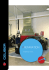### FleetValue ™ Oil Filters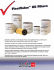### MADE IN THE USA - FreshWaterSystems.com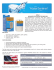### Airscreen 1000 14 x 24 Brochure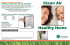### Greater Cincinnati Water Works Filter Distribution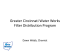### Extend Lube Filter life and reduce operating cost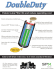### Air filters with a HEPA filter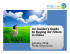### 570-491 EZflex:Layout 1.qxd